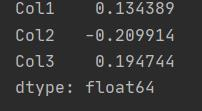Loading, please wait...# Function Application in Pandas | pipe() | apply() | applymap()

Feb 22, 2021 apply() , pipe() , applymap(), 4293 Views

Sometime user wants to apply their own functions or another library’s function to pandas objects. So, through this blog we will learn about those methods. The appropriate method to use depends on whether your function expects to operate on the entire DataFrame, row r column-wise, or element-wise.

# Function Application in Pandas

•    Table wise Function Application: pipe()
•    Row or Column wise Function Application: apply()
•    Element wise Function Application: applymap()

Now, we will how these methods are applied on the pandas' object i.e DataFrame.

### a. Table wise Function Application: pipe():

If you want to apply the function table-wise, then perform the custom operation bypassing the function and the appropriate number of parameters as the pipe arguments. Thus, the operation is performed in the whole pandas' object i.e DataFrame.

Now we will use the custom function to conduct the operation on the DataFrame.
Example:

Code:

``````import pandas as pd
import numpy as np

return element1+element2

df = pd.DataFrame(np.random.randn(10, 3), columns=['Col1', 'Col2', 'Col3'])
print(df)

print(df1)``````

Code Explanation :
Import libraries and then Create a function that you want to apply to the data frame, here I am creating an adder() function, it will take two elements as an argument, and then using NumPy library create a table with any number of rows and columns. At last, we will use the pipe() method for table-wise function application.
Output: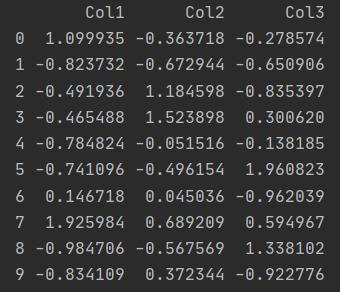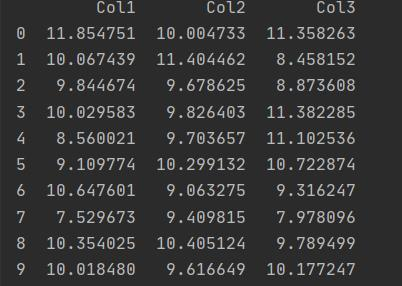b. Row or Column wise Function Application: apply()

If you want to apply some arbitrary functions along the axis of the panel or DataFrame, you can use apply() methods, which, like the descriptive statistics methods,  takes an optional axis argument.

Example 1: Code to perform Column wise operation
Code:

``````import pandas as pd
import numpy as np

return element1+element2

df = pd.DataFrame(np.random.randn(10, 3), columns=['Col1', 'Col2', 'Col3'])
print(df)
print(df1)
print(df1.apply(np.mean))
``````

Output: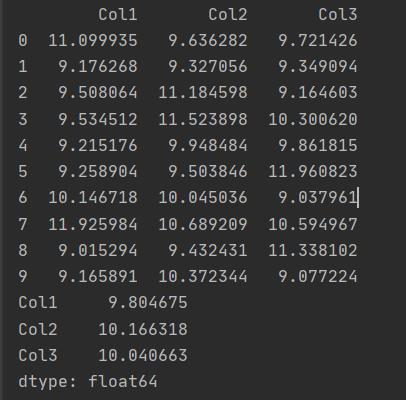Example 2: Code to perform Row wise operation
Code:

``````import pandas as pd
import numpy as np

df = pd.DataFrame(np.random.randn(10, 3), columns=['Col1', 'Col2', 'Col3'])
print(df)

print(df1.apply(np.mean, axis=1))``````

Output: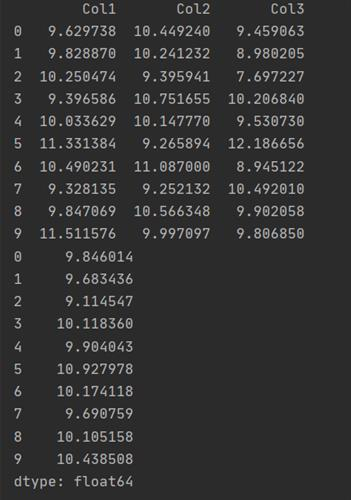Example 3: Code to perform lambda function on apply method
Code:

``````import pandas as pd
import numpy as np

df = pd.DataFrame(np.random.randn(10, 3), columns=['Col1', 'Col2', 'Col3'])
#My Custom Function
df['Col1'].map(lambda x:x+10)
print(df.apply(np.mean))``````

Output: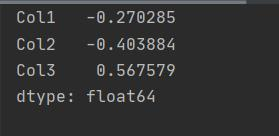c. Element wise Function Application: applymap()

Example1: Code to perform the element-wise operation

Code:

``````import pandas as pd
import numpy as np

df = pd.DataFrame(np.random.randn(10, 3), columns=['Col1', 'Col2', 'Col3'])
#My Custom Function
df.applymap(lambda x:x+10)
print(df.apply(np.mean))``````

Output: